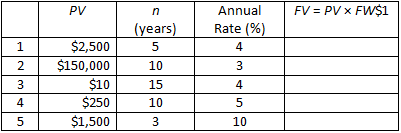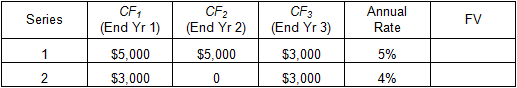Time Value of Money - Six Functions of a Dollar
Using Assessors Handbook Section 505 (Capitalization Formulas and Tables)
Appraisal Training: Self-Paced Online Learning Session

# Lesson 2: Future Worth of \$1

In order to solve the following problems you will need to refer to AH 505 to look up the factors in the compound interest tables. Before you start, please print the problems using the "Print Questions" button below so you can work through the problems on your own. After you work through the problem, the solution can be viewed by clicking on the blue plus sign immediately following the question. Additionally, when you have completed all the problems, you may print all of the solutions using the "Print Questions with Answers" button below.

## Problem 1

Calculate the future value (FV) of each present value (PV) listed below using the future worth of \$1 (FW\$1) factors in AH 505:## Problem 2

You have a choice of investing in either of two accounts:

• Account A earns an annual rate of 5% with annual compounding
• Account B earns an annual rate of 5.25% at simple interest

You plan to invest \$10,000 in one of the accounts today and withdraw the funds 4 years later. In which account should you place your funds?

## Problem 3

If you deposit \$10,000 in an account that pays an annual interest rate of 7%, how much money will you have at the end of:

1. 10 years?
2. 20 years?
3. 40 years?

## Problem 4

You deposit \$10,000 today in an account that earns an annual rate of 7.5%. At the end of 5 years, how much interest on interest will the account have earned? Hint: The amount of interest on interest is the difference between the future value at compound interest and the future value at simple interest.

## Problem 5

You have just purchased an investment property for \$400,000. You expect the value of the property to increase at an annual compound interest rate of 8% over the next 10 years. How much do you expect the property to be worth after 10 years?

## Problem 6

Assuming all cash flows (CF) occur at the end of each year, determine the future values of each of the following two series of cash flows: